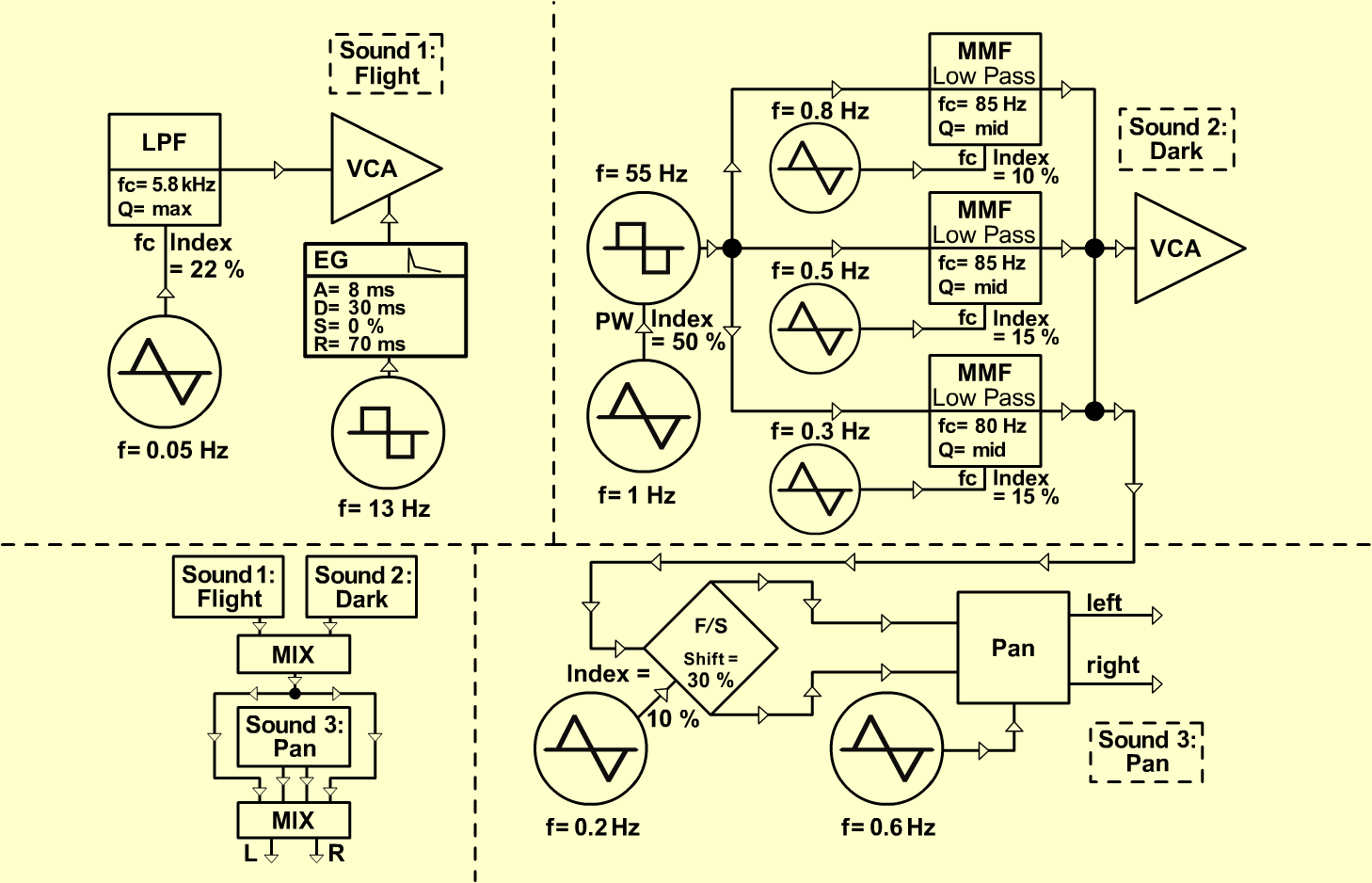The FlightTextureD o e p f e r A - 100 Connections: Settings: Sound 1 (Flight): A-145/1 (Triangle)  <=>  A-120 (CV 3) A-120 (Audio Out)  <=>  A-131/1 (Audio In) A-145/2 (Pulse)  <=>  A-140 (Gate) A-140 (Output)  <=>  A-131/1 (CV 1) A-131/1 (Audio Out)  <=> Mixer 1 Sound 2 (Dark): A-111 (Pulse)  <=>  A-127 (Audio In) A-145/3 (Triangle)  <=>  A-111 (PCV) A-127 (Mix)  <=>  A-131/2 (Audio In) A-131/2 (Audio Out)  <=> Mixer 2 Sound 3 (Pan): A-127 (VCF 3)  <=>  A-126 (Audio In) A-145/4 (Triangle)  <=>  A-126 (CV 1) A-126 (Down Output)  <=>  A-134 (Audio In 1) A-126 (Up Output)  <=>  A-134 (Audio In 2) A-145/5 (Triangle)  <=>  A-134 (CV 1) A-134 (Left Output)  <=> Mixer 3 A-134 (Right Output)  <=> Mixer 4 A-120 (Freq = 7, CV 3 = 2.2, Lev = 10, Res = 10) A-145/1 (Frq = 1, Range = L) A-145/2 (Frq = 8, Range = M) A-140 (A = 1, D = 1, S = 0, R = 1, Range = M) A-131/1 (Gain = 0, Audio In 1 = 10,  Audio Out = 10) A-111 (Octave = -1, PW = 5, PCV = 5) A-145/3 (Frq = 7.7, Range = L) A-127 (Audio In = 8, Original = 0; Row 1: LFO Freq = 2.5, CV Ampl = 1, VCF Frq = 3, Res = 6.5, Audio Level = 10; Row 2: LFO Freq = 2, CV Ampl = 1.5, VCF Frq = 3, Res = 6.5, Audio Level = 10; Row 3: LFO Freq = 1.5, CV Ampl = 1.5, VCF Frq = 2.5, Res = 4.5, Audio Level = 10) A-131/2 (Gain = 10, Audio In 1 = 10,  Audio Out = 10) A-126 (Level = 7, Shift = 3, CV = 1) A-145/4 (Frq = 4, Range = L) A-134 (Pan = 5, Lev 1 = 10, Lev 2 = 10) A-145/5 (Frq = 7.2, Range = L) Notes: Frequency of A-111: 55 Hz. A-111: The modul A-110 is also possible. A-145: If you don't have five A-145-Modules, use A-146, A-147, the LFOs from A-190 or MCV24, a low tuned A-110 (> 8 Hz for A-145/3). Mixer 1 - 4 with A-100-Modules:   Mixer 1  <=>  A-138/1 (Input 1)   Mixer 2  <=>  A-138/1 (Input 2)   A-138/1 (Output)  <=>  A-180   A-180  <=>  A-138/2 (Input 1)   A-180  <=>  A-138/3 (Input 1)   Mixer 3  <=>  A-138/2 (Input 2)   Mixer 4  <=>  A-138/3 (Input 2)   A-138/2 (Output)  <=> Amplifier (Left)   A-138/3 (Output)  <=> Amplifier (Right) Axel Jungkunst, April 2001Sound sample The Flight (with dig. Delay)